StatLect

# Information matrix

The information matrix (also called Fisher information matrix) is the matrix of second cross-moments of the score vector. The latter is the vector of first partial derivatives of the log-likelihood function with respect to its parameters.## Definition

To define the information matrix, we need the following objects:

• a sample;

• aparameter vectorthat characterizes the distribution of;

• the likelihood function;

• the log-likelihood function• the score vectorthat is, thevector of first derivatives ofwith respect to the entries of.

The information matrixis thematrix of second cross-moments of the score:The notationindicates that the expected value is taken with respect to the probability distribution ofassociated to the parameter.

## The expected value

We take an expected value because the sampleis random.

For example, if the samplehas a continuous distribution, then the likelihood function iswhereis the probability density function of, parametrized by.

Then, the information matrix is## The information matrix is the covariance matrix of the score

Under mild regularity conditions, the expected value of the score is equal to zero:As a consequence,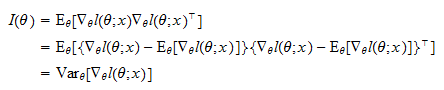that is, the information matrix is the covariance matrix of the score.

## Information equality

Under mild regularity conditions, it can be proved thatwhereis the matrix of second-order cross-partial derivatives (so-called Hessian matrix) of the log-likelihood.

This equality is called information equality.

## Example: information matrix of the normal distribution

As an example, consider a samplemade up of the realizations ofIID normal random variables with parametersand(mean and variance).

In this case, the information matrix isProof

The log-likelihood function is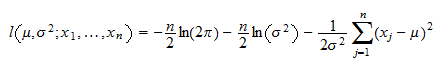as proved in the lecture on maximum likelihood estimation of the parameters of the normal distribution. The scoreis avector whose entries are the partial derivatives of the log-likelihood with respect toand:The information matrix is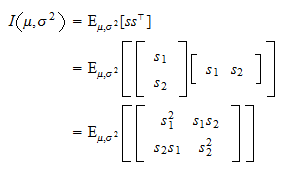We havewhere: in stepwe have used the fact that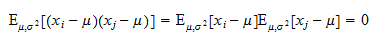forbecause the variables in the sample are independent and have mean equal to; in stepwe have used the fact thatMoreover,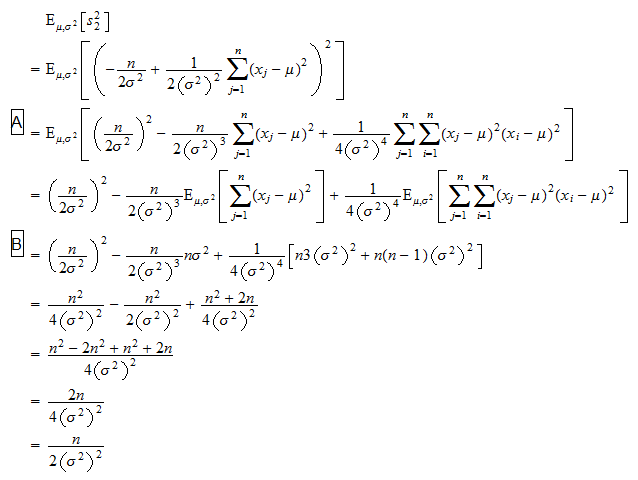where: in stepsandwe have used the independence of the observations in the sample and in stepwe have used the fact that the fourth central moment of the normal distribution is equal to. Finally,where: in stepwe have used the facts thatand that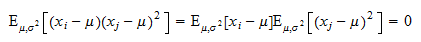forbecause the variables in the sample are independent; in stepwe have used the fact that the third central moment of the normal distribution is equal to zero.

## Covariance matrix of the maximum likelihood estimator

When the sampleis made up of IID observations, as in the previous example, the covariance matrix of the maximum likelihood estimator ofis approximately equal to the inverse of the information matrix.

Denote the maximum likelihood estimator ofby. Then,Proof

Denote bytheiid observations. The log-likelihood of the sample iswhereis the log-likelihood of the-th observation. Under some technical conditions, we have proved thatconverges in distribution to a normal distribution with zero mean and covariance matrix equal toThis implies thatwhere: in stepwe use the fact that the observations are identically distributed; in stepwe can bring the summation inside the variance operator because the observations are independent; in stepwe exploit the linearity of the gradient; in stepwe use the fact that the information matrix is equal to the covariance matrix of the score.

Note that in general, this is true only if the observations in the sample are independently and identically distributed.

## More details

More details about the Fisher information matrix, including proofs of the information equality and of the fact that the expected value of the score is equal to zero, can be found in the lecture on Maximum likelihood.

## Keep reading the glossary

Previous entry: Impossible event

Next entry: Integrable random variable